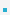1. Definitions Define some words as follows:Datatypes: – The datatype of an object in memory determines the set of values it can have and what operations    that can be performed on it. – C is a weakly typed language. It allows implicit conversions as well as forced (potentially dangerous)    casting.Operators: – Operators specify how an object can be manipulated (e.g.,, numeric vs. string operations). – operators can be unary(e.g., -,++),binary (e.g., +,-,*,/),ternary (?:)Expressions: – An expression in a programming language is a combination of values, variables, operators, and    functionsVariables: – A variable is as named link/reference to a value stored in the system’s memory or an expression that    can be evaluated. Consider: int x=0,y=0; y=x+2;. where x, y are variables, y = x +2 is an expression, and + is an operator.

 이전페이지 / 2 / 다음페이지